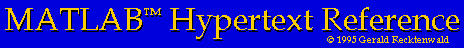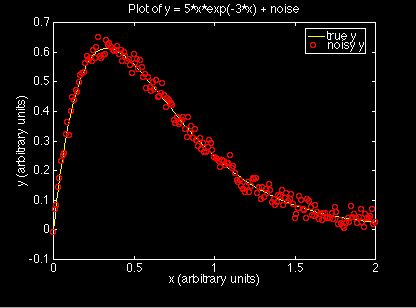# Putting Multiple Curves on the Same Plot

This page describes how to plot y1 = f1(x), y2 = f2(x), ... yn = fn(x) on the same plot, and how to label these curves with the legend command.

## Passing multiple data pairs to the `plot` command

The plot command will put multiple curves on the same plot with the following syntax
```	plot(x1,y1,s1,x2,y2,s2,x3,y3,s3,...)
```
where the first data set (x1,y1) is plotted with symbol definition s1, the second data set (x2,y2) is plotted with symbol definition s2, etc. The separate curves are labeled with the legend command as demonstrated in the following example.

Note that x1, x2, ... etc need not be the same vector.

Each pair of vectors, (x1,y1), (x2,y2), etc. must have the same length. In other words the length of x1 and y1 must be the same. The length of x2 and y2 must be the same, but can be different from the length of x1 and y1.

## The `legend` command

The `legend` is used to label the different data curves when more than one curve is drawn on the same plot. The syntax is
```	legend('label1','label2',...)
```
Where label1, etc are strings used to create the legend. This is best demonstrated by an example, which follows.

## An example of putting multiple curves on a plot

Here are the MATLAB commands to create a symbol plot with the data generated by adding noise to a known function. The original function is drawn with a solid line and the function plus noise is plotted with open circles. These commands are also in the script file noisyDataLine.m for you to download.

```	>> x = 0:0.01:2;                 %  generate the x-vector
>> y = 5*x.*exp(-3*x);           %  and the "true" function, y
>> yn = y + 0.02*randn(size(x)); %  Create a noisy version of y
>> plot(x,y,'-',x,yn,'ro');      %  Plot the true and the noisy

>> xlabel('x (arbitrary units)');       %  add axis labels and plot title
>> ylabel('y (arbitrary units)');
>> title('Plot of y = 5*x*exp(-3*x) + noise');
>> legend('true y','noisy y');

```
Note that the ``.*'' operator is necessary when multiplying the vector `x` by the vector `exp(-3*x)`. The preceding statments create the following plot.